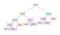# Solving Fibonacci Numbers using Dynamic Programming

Dynamic programming is a method for solving a complex problem by breaking it up into smaller subproblems, and store the results of the subproblems for later use (to reduce duplication).

This article on GeeksforGeeks explains:

Wherever we see a recursive solution that has repeated calls for same inputs, we can optimize it using Dynamic Programming.

Let’s start with the Fibonacci numbers.

The Fibonacci numbers is the sequence `0, 1, 1, 2, 3, 5, 8, 13, 21, 34 …` where each number in the sequence is found by adding up the two numbers before it.

Note: It is sometimes written `1, 1, 2, 3, 5, 8, 13, 21, 34 …` but we will be using the sequence above where `fib(0) = 0`.

Let’s write a function that will return the nth Fibonacci number.

`function fibonacciNoRecursion(n){  if (n < 0) return undefined;  if (n === 0) return 0;  let previous = 1;  let sum = 1;  for (let i = 2; i <= n; i++){    let temp = sum;    sum += previous;    previous = temp;  }  return sum}`

This has a linear time complexity —` O(n)`.

The recursive solution is more elegant:

`function fib(n){  if (n < 0) return undefined;  if (n < 2) return n;  return fib(n-1) + fib(n-2)}`

but its time complexity is exponential, or `O(2^n)`, which is not ideal at all.

There are the two indicators that dynamic programming can be utilized to solve a specific problem: overlapping subproblems and optimal substructure. We will explain what they are and demonstrate that finding the nth Fibonacci number is a good example of both.

## Overlapping Subproblems

This article on educative.io explains:

Subproblems are smaller versions of the original problem. Any problem has overlapping sub-problems if finding its solution involves solving the same subproblem multiple times. Take the example of the Fibonacci numbers; to find the `fib(5)`, we need to break it down into the following sub-problems:https://www.slitherintopython.com/book/chapter_16/chapter_16.html

Using the color-coded image above, we can see just how many overlapping subproblems there are. To solve for `fib(5)`, we need to calculate `fib(3)` twice, `fib(2)` three times etc. This is duplication that we would like to eliminate.

## Optimal Substructure

Optimal substructure means an optimal solution can be constructed from optimal solutions of its subproblems.

Fibonacci of n is defined as follows:

`fib(n) = fib(n-1) + fib(n-2)`

The optimal solution for n depends on the optimal solution of `(n-1)` and `(n-2)`.

There are two ways to solve the Fibonacci problem using dynamic programming.

## 1. Memoization

Memoization stores the result of expensive function calls (in arrays or objects) and returns the stored results whenever the same inputs occur again. In this way we can remember any values we have already calculated and access them instead of repeating the same calculation.

This is a top-down approach, meaning we start with what we are trying to find. We start from `fib(5)` and work our way down, filling in the gaps and adding them all together. (To to find `fib(5)` we calculate `fib(4)` and `fib(3)` etc. )

`function memoizedFib(n, memo={}){  if (memo[n]){    return memo[n];  }  else if (n === 0 || n === 1){    return 1;  }  let result = memoizedFib(n-1, memo) + memoizedFib(n-2, memo);  memo[n] = result;  return result;}`

## 2. Tabulation

Tabulation is usually accomplished through iteration (a loop). Starting from the smallest subproblem, we store the results in a table (an array), do something with the data (for example: add the data for Fibonacci) until we arrive at the solution.

This is a bottom-up approach. We start from the bottom, finding `fib(0)` and `fib(1)`, add them together to get `fib(2)` and so on until we reach `fib(5).`

`function tabulatedFib(n){  if (n === 1 || n === 2){    return 1;  }  const fibNums = [0, 1, 1];  for (let i = 3; i <= n; i++){    fibNums[i] = fibNums[i-1] + fibNums[i-2];  }  return fibNums[n];}`

The time complexity of both the memoization and tabulation solutions are `O(n)` — time grows linearly with the size of n, because we are calculating `fib(4)`, `fib(3)`, etc each one time.

Note: While both have the linear time complexity, since memoization uses recursion, if you try to find the Fibonacci number for a large `n`, you will get a stack overflow (maximum call stack size exceeded).

Tabulation, on the other hand, doesn’t take as much space, and will not cause a stack overflow.

Another note: Fibonacci numbers may be a flawed example for a couple of reasons. A number large enough to cause a stack overflow with memoization is a solution that is too large for Javascript to give the accurate result for. Second, our original solution had linear time complexity and constant space complexity (without recursion or dynamic programming). In any case, dynamic programming is an important concept to learn and perhaps Fibonacci numbers is used since it is a simple example and it makes dynamic programming easier to understand.

References:

Software Engineer | Full Stack Developer

## More from Elisheva Elbaz

Software Engineer | Full Stack Developer